# Functional Currency Decimals

Dynamics GP allows setting the Currency Decimal Places on an Item to a larger number than the Company’s Functional Currency Decimal Places (FCDP)  For example, an Item could have 5 currency decimals (\$12.00124) but the FCDP (which governs the General Ledger) only allows 2 currency decimals (\$12.00).  What happens when you add inventory to the system with this sort of setup?  Read on to hear the Halloween Story of the Disappearing Inventory Value.

As mentioned above, this tale starts with Dynamics GP when the Functional Currency Decimal Places is set to 2 and Item Currency Settings are more than 2.

Item TEST6 has 5-decimals. The rest of the story uses this 5-decimal Item because it exaggerates the loss of value.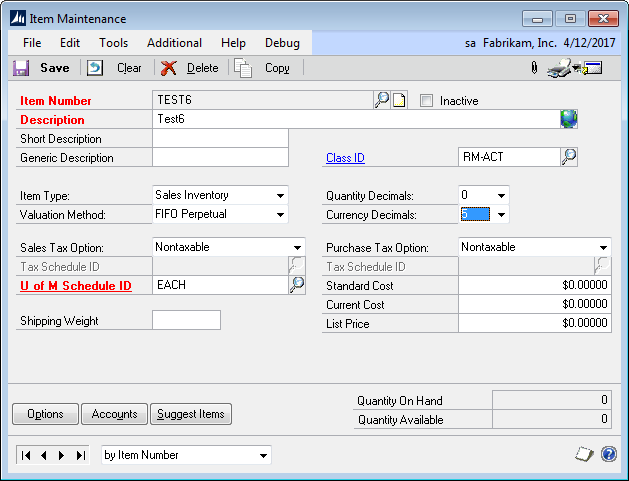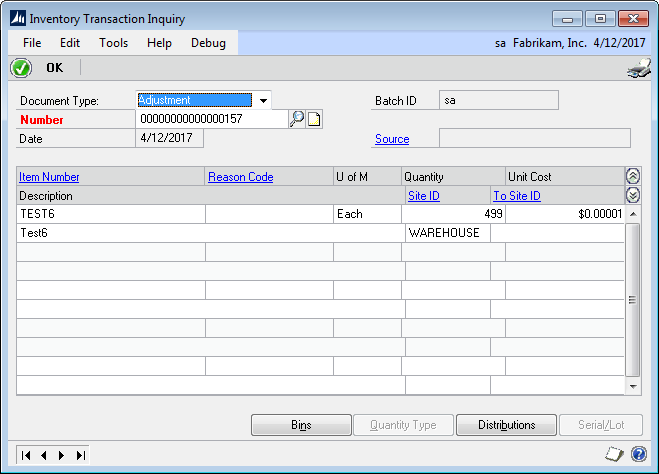The IV Transaction Line table in the SQL database (shown below) has the correct unit cost, but GP has already rounded the Extended Cost. The value that should be in EXTDCOST is \$0.00499 (which is the quantity of 499 multiplied by the cost of \$0.00001).  Since the FCDP is 2, GP looks at the 3rd decimal place value and rounds. In this case it rounds to zero. So the extended cost is zero.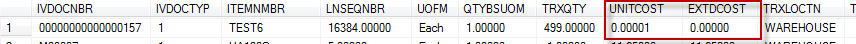The Inventory Posting Journal reflects this: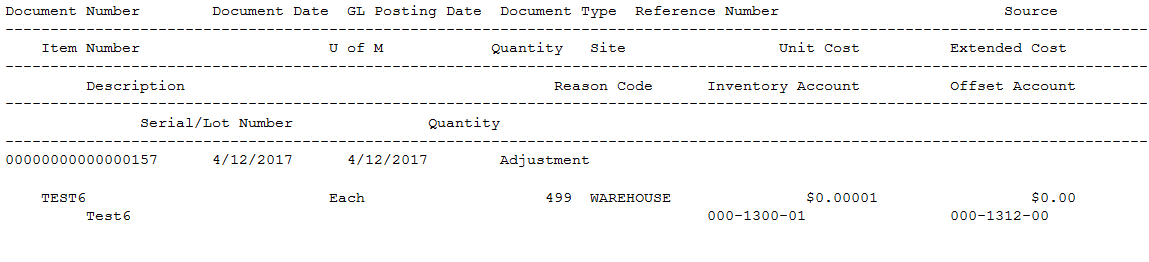And the GL Distribution Register is all zeros: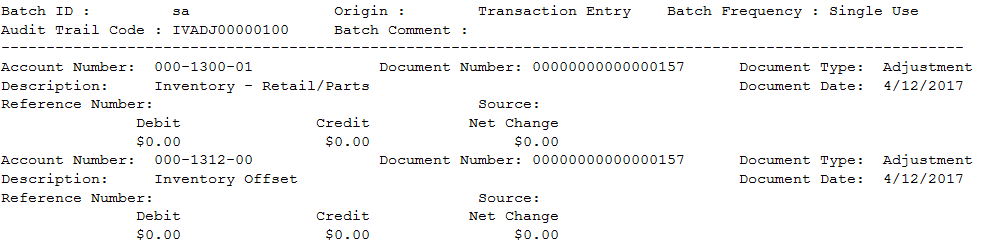The Purchase Receipt Layer (or “FIFO Layer”) shows the correct quantity, but it too was added at the rounded cost:No Journal Entry is created because GP sees this as a zero-dollar transaction.

The same situation occurs with Purchase Orders. Although you can enter a Unit Cost to five decimals, it is immediately rounded to 2-decimals in the Extended Cost, which results in zero dollars.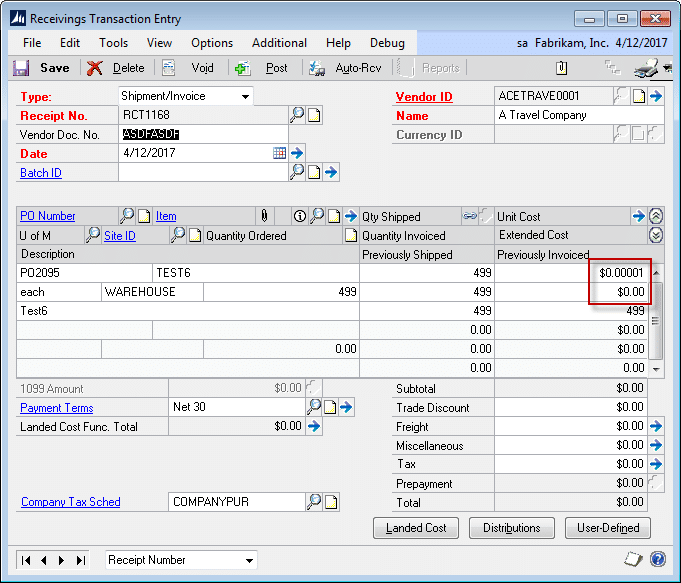This can also be seen in the PO Line table: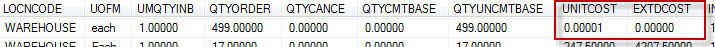The PO Receipt shows the correct Unit Cost, but Extended Cost is rounded to zero.When the PO Receipt is posted, just like the Inventory Adjustment, it creates a Purchase Receipt Layer with the correct quantity, but zero dollars.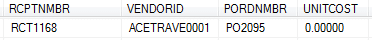Item Transaction Inquiry shows the two transactions with the correct Unit Cost. With two receipts of the item, there should now be 998 * \$0.00001 = \$0.00998 in inventory, but neither of these transactions hit the GL, so the inventory account would still show \$0.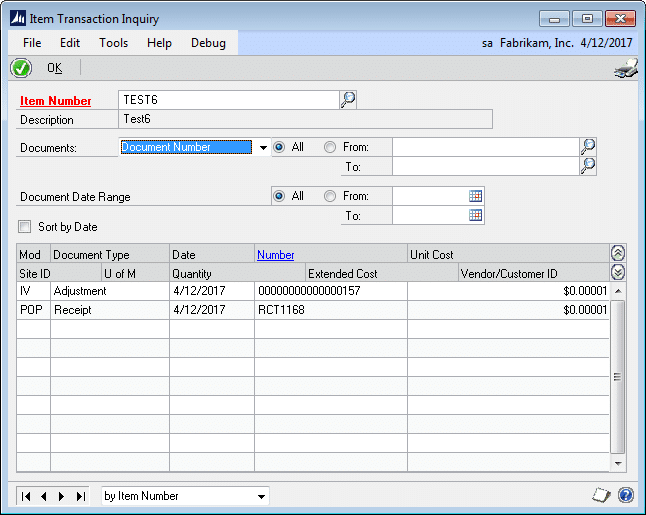Using \$0.00001 highlights the effect, but the same thing occurs when the actual inventory value is \$523.55449. In this case you lose \$0.00449 because the transaction gets rounded to \$523.55.

In theory, over a very large number of transactions, there might be enough rounding up and down that the effect averages out. However, it is certainly plausible that you have a manufacturing process which creates a large number of transactions that all lose a significant amount of value.

Avoid the Ghost of Lost Value by avoiding the setup described above.  Make sure the Item Currency Decimals setting does not exceed the FCDP setting.